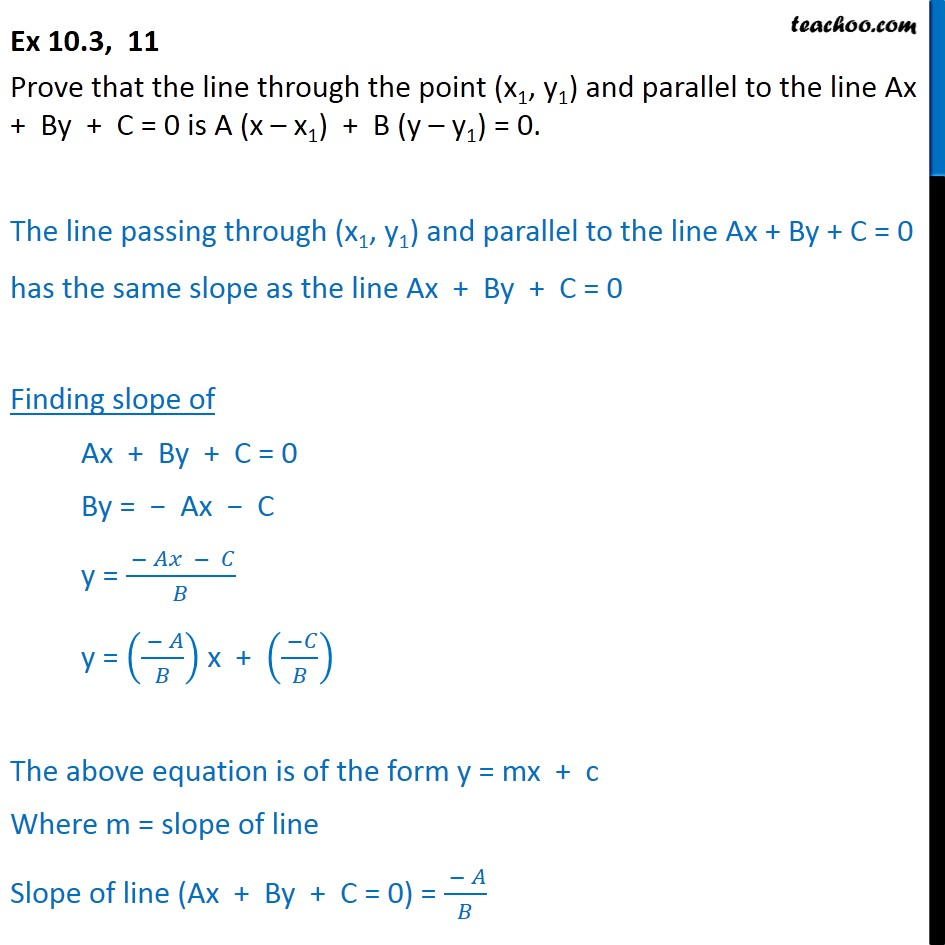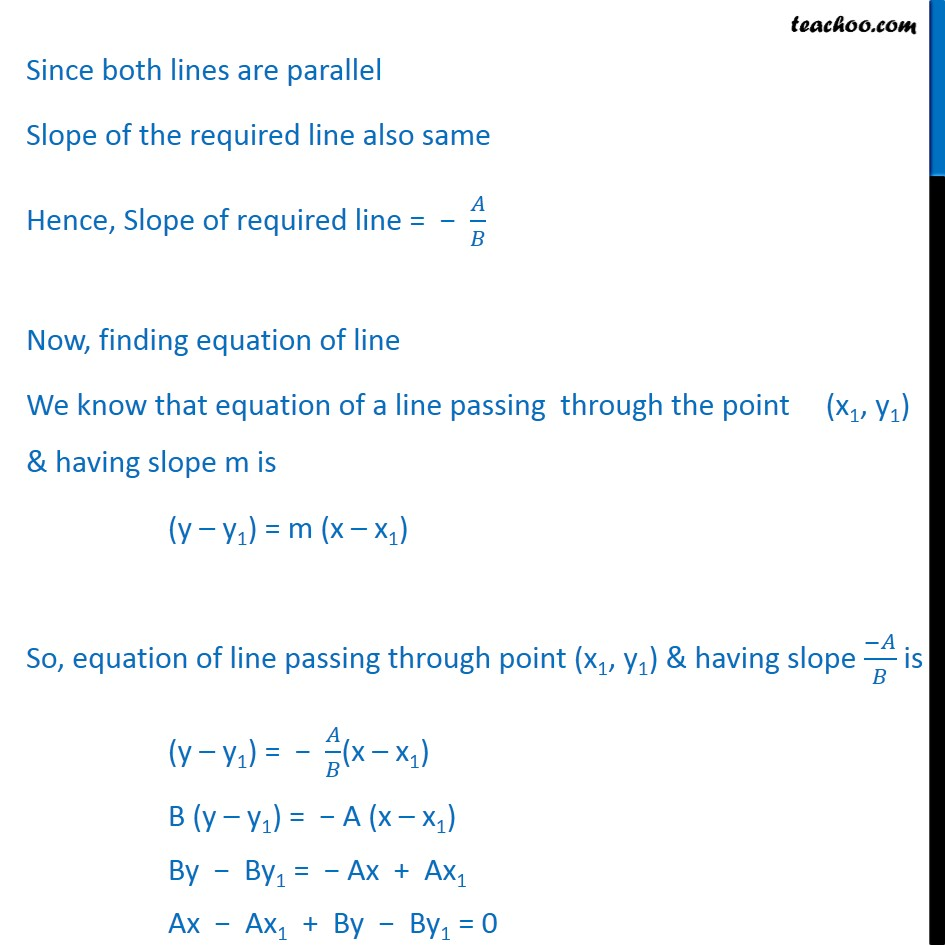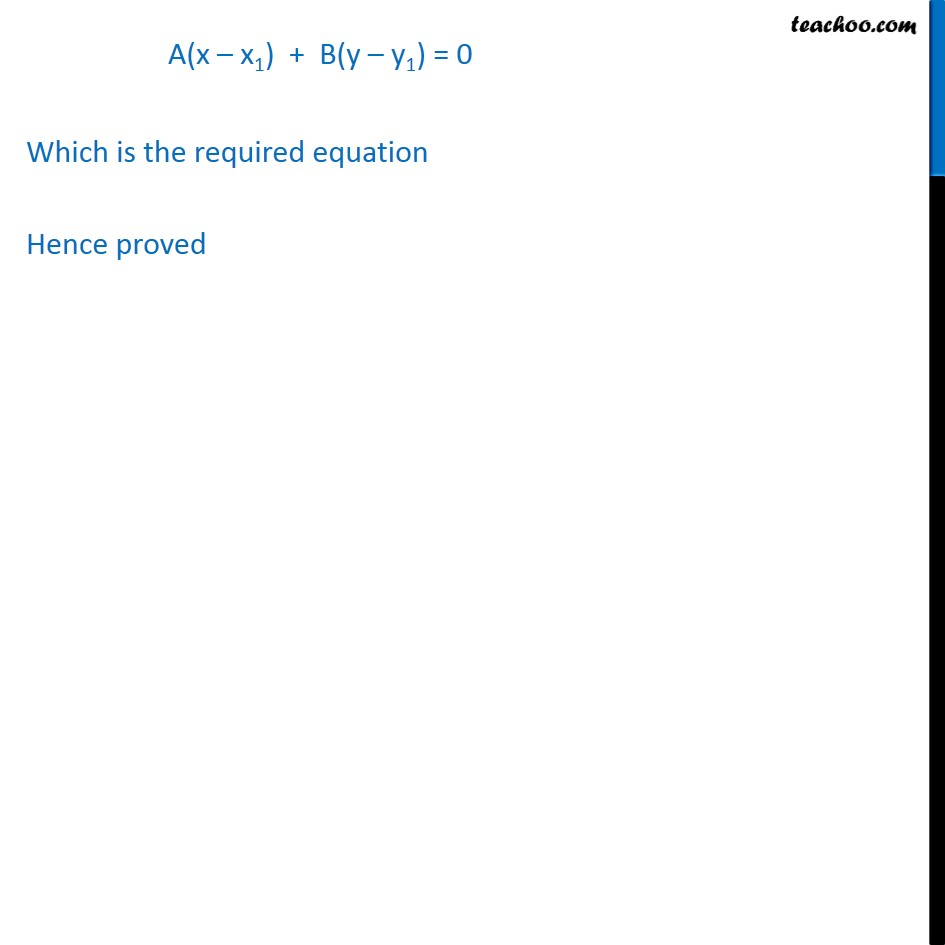Ex 10.3

Chapter 10 Class 11 Straight Lines (Term 1)
Serial order wise### Transcript

Ex 10.3, 11 Prove that the line through the point (x1, y1) and parallel to the line Ax + By + C = 0 is A (x – x1) + B (y – y1) = 0. The line passing through (x1, y1) and parallel to the line Ax + By + C = 0 has the same slope as the line Ax + By + C = 0 Finding slope of Ax + By + C = 0 By = − Ax − C y = ( − 𝐴𝑥 − 𝐶)/𝐵 y = (( − 𝐴)/𝐵) x + (( −𝐶)/𝐵) The above equation is of the form y = mx + c Where m = slope of line Slope of line (Ax + By + C = 0) = ( − 𝐴)/𝐵 Since both lines are parallel Slope of the required line also same Hence, Slope of required line = − 𝐴/𝐵 Now, finding equation of line We know that equation of a line passing through the point (x1, y1) & having slope m is (y – y1) = m (x – x1) So, equation of line passing through point (x1, y1) & having slope (−𝐴)/𝐵 is (y – y1) = − 𝐴/𝐵(x – x1) B (y – y1) = − A (x – x1) By − By1 = − Ax + Ax1 Ax − Ax1 + By − By1 = 0 A(x – x1) + B(y – y1) = 0 Which is the required equation Hence proved